## Time Functions and Formulas in Excel (part3)

Here some more of the very important date and time function of Excel. Weekdays, Now, Years are discussed here.

Look at part 1 for Date here.

Part 2 deals with Time, hours, minutes functions and formulas

Two very useful functions are the NETWORKDAYS and WORKDAY to calculate difference between date without the weekends and holidays as well as find the next working day after a defined date. These are part of the top 10 functions in Excel. Find an explanation in our top 10 page.

### WEEKDAY

This functions is very useful as it gives you which day (Monday to Sunday) a certain date is.

Actually, it does not give you the name of day but a number representing it (1 to 7).

It is used simply by typing =WEEKDAY("4/5/2015") which results in 2 (which is a Monday)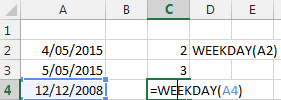Then you have the option to change which day is represented by which number.  Like you want Monday to be 1, Tuesday to be 2, ...

Then you have to type =WEEKDAY("4/5/2015",2) which results in 1 (which is a Monday)

Of course you can put a cell instead of the "4/5/2015", like D2   =WEEKDAY(D2 , 2)

Here the the correspondance table for the optional day selection. The most used are of course 1,2,3.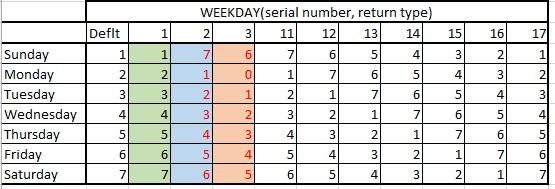### NOW(): shows you the time.

The Now() function returns the current day and the current time.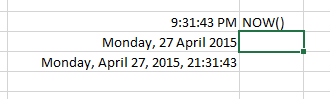It is very useful when you want to show your user this information.

TIP: a useful trick is to type  CTRL ;   This will give you the day, and CTRL : will display the time in the cell. But these values will be fixed and not updating like NOW or TODAY().

TIP: to update the time, press F9.

### WEEKNUM(): gives you the weeknumber

This very useful to indicate the weeknumber of a date.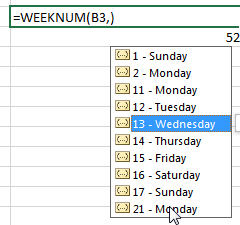### YEARFRAC: gives you the fraction of the year between two dates

YEARFRAC is a useful function to rapidely calculated the fraction of the year between two dates.

For example you want to know what fraction of the year is between 1 march and 31 mai. YEARFRAC("1/3/2015", "31/5/2015")  gives you exactly 0.25 or a quarter of a year.

Here some examples from january first.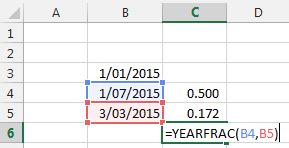You can select the system you want to be in. For example a 30 days per month system that is common in the financial and accounting industry (they need to make things simples (;-) ) or based on a real year.Here you will find listed all the Date and Time functions so that you can have a good overview. In Bold are the ones most used and useful.

So that's it for the Date and Time Formulas and Functions in Excel.

Now, let's use them....